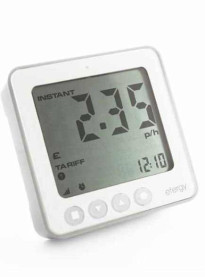# Accuracy of Wireless Power Metering

Wireless "power" meters like the Efergy don't actually measure power. To keep their connection simple and cost down, they only measure peak current and they make three assumptions that are not always warranted and that lead them to overestimate the power.

1. They assume the RMS voltage is 230 V (or whatever voltage you tell them).
2. They assume the current waveform is sinusoidal and so RMS current is peak current divided by the square root of 2.
3. They assume the current waveform is in phase with the voltage waveform and so power is RMS current times RMS voltage.

Assumption 1 is usually not a great source of error, but 2 and 3 can be, and are both often described as the meter ignoring "power factor".

Assumption 2 can overestimate power by a huge amount in the case of some low powered electronic devices and is likely what's happening here. The appliance only draws current in a very short "spike" at the peaks of the voltage waveform, and the "power" meter assumes the peak value of this is the peak of a sine wave of current.

Assumption 3 can overestimate power by up to 40% in the case of loads which are motors, such as pumps. A common "phantom" is a solar hot water system circulating pump for split systems (i.e. where the tank is not on the roof). A power factor correcting capacitor can be installed across these. This won't save any power, but will at least make the wireless "power" meter read more nearly correct.

The only way to know is to use a proper power meter that simultaneously measures voltage and current and multiplies their instantaneous values together, making none of the above assumptions. But these are more difficult to wire in, in the case of a permanently wired appliance, and this should only be done by an electrician.